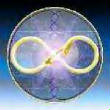# Edward Leedskalnin - Coral CastleGuest
Guest

note: (re)move if not in the right place or alredy published

Explained via prime numbers and geometry

1 of 6 (search for the rest on youtube)

http://www.code144.com/Didymos

Posts : 795
Join date : 2010-05-20
Location : Queanbeyan, NSW, Australia

Timemarker 5:22-5:30 is in error as the precise
Phi=½[√5+1]=1.618033989...=2sin(18°)

and not the repeating fraction

44.5/27.5=89/55=1.6181818... as stated at the timemarker.
Even more precisely, the Fibonacci series is in fact mathematically defined as adjacent relative prime terms in:
0,1,1,2,3,5,8,13,21,34,55,89,144,...

So this validates Ed Leedskalnin's and the author's discovery indeed.
He should however emphasise that the prime number quadrupoles are a consequence of established Prime Number Theory, say as encountered in the analysis of the Riemann Hypothesis (incorporating the Prime Number Theorem where the Number of Primes in a Natural Number Count N is approximated by the ratio N/lnN - so the first 100 Natural Numbers 1,2,3...,99,100 should accomodate 100/ln100~21.7=22 as a first approximation (the true value is 25 so it is a 'bad' first approximation).

Just as the Fibonacci series diverges rapidly, because the 'distance' between the Natural Numbers increases faster than a linear counting parameter; so does the Prime Number Theorem becomes more and more accurate as the Prime Numbers get larger (and the difference of 4 between the central qaudrupoles becomes rarer with the difference of two difference adjacents:
5-7-11-13=5-(7-(4)-11)-13
11-(13+4=17)-19......101-(103+4=107)-109......191-(193+4=197)-199......etc

Overall however, excellent work and truly the discovery of Ledskalnin's 'Magnetic Current' which is in fact the Monopolic Masscurrent of modern membrane stringphysics.

The possible application of this monopolar stringforce as an antigravitational agency at Coral Castle (as is the reported phenomena of Easter Island Statues and the manouvers of Tibetean monks using sound) appears however to have been an isolated one-to-one case of the Newtonian Forcelaw extension of the
Unified Field's Alpha-Force: F=ma=d(p)/dt=d(mv)/dt=mdv/dt+v.dm/dt with relativistic mass m=hf/c².

Then in the basic linear coordinate vector case:
F=ma becomes extended in F=mdv/dt (classical and known)+hv/c².df/dt (Falpha and relatively unknown because the (coefficient) factor hv/c²~10^-42 and classically unmeasurable).

Therefore the Awareness df/dt=Consciousness Acceleration must become extremely HIGH to overpower the small classical term of the coefficient.
It is possible though, because the maximum entropy count of the timedifferental for frequency is the square of the wormhole resonance and so 9x10^60.

Later on the Pyramid angle is a simple geometry as the complementary angle of the pyramids sideangle of 51.83 degrees is of course 90-58.83=38.17 degrees and the angle, any angle of sin(38.17 degrees)=0.61800.. which again APPROXIMATES 1/PHI=0.618033...
The precise pyramid side angle so should be exactly 90-38.17270762... degrees for perfect geometric alignment in 51.82729238..degrees.

Tonyblue

Current date/time is Sun Jun 07, 2020 2:15 am